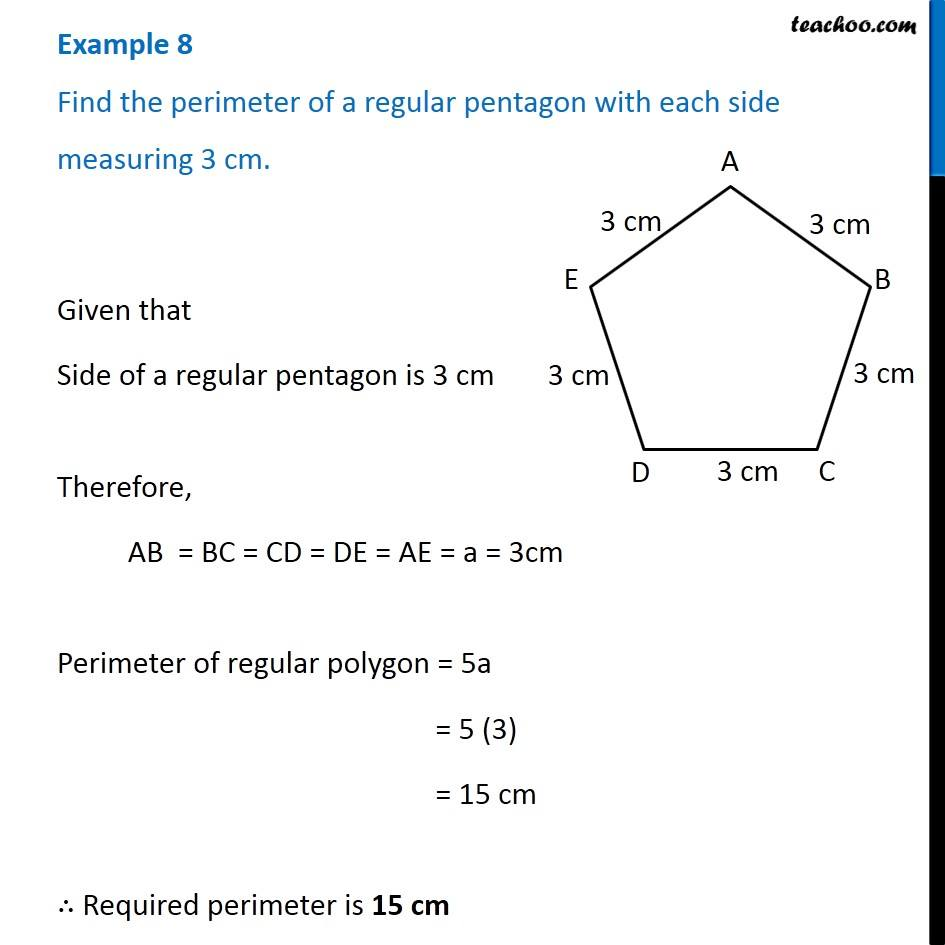Examples

Chapter 10 Class 6 Mensuration
Serial order wiseLearn in your speed, with individual attention - Teachoo Maths 1-on-1 Class

### Transcript

Example 8 Find the perimeter of a regular pentagon with each side measuring 3 cm. Given that Side of a regular pentagon is 3 cm Therefore, AB = BC = CD = DE = AE = a = 3cm Perimeter of regular polygon = 5a = 5 (3) = 15 cm ∴ Required perimeter is 15 cm